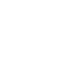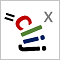# CLIJ2GPU accelerated image processing for everyone

CLIJ2 home

## cylinderTransformApplies a cylinder transform to an image stack assuming the center line goes in Y direction in the center of the stack.

This transforms an image stack from an XYZ coordinate system to a AYD coordinate system with A the angle around the center line, Y the original Y coordinate and D, the distance from the center.

Thus, going in virtual Z direction (actually D) in the resulting stack, you go from the center to theperiphery.

### Usage in ImageJ macro

``````Ext.CLIJx_cylinderTransform(Image input, Image destination, Number number_of_angles, Number delta_angle_in_degrees, Number relative_center_x, Number relative_center_z);
``````

Imprint